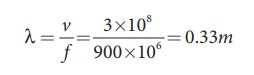Home | | Physics 11th std | Particle velocity and Progressive wave velocity

# Particle velocity and Progressive wave velocity

In a plane progressive harmonic wave, the constituent particles in the medium oscillate simple harmonically about their equilibrium positions.

Particle velocity and wave velocity

In a plane progressive harmonic wave, the constituent particles in the medium oscillate simple harmonically about their equilibrium positions. When a particle is in motion, the rate of change of displacement at any instant of time is defined as velocity of the particle at that instant of time. This is known as particle velocity.Similarly, we can define velocity (here speed) for the travelling wave (or progressive wave). In order to determine the velocity of a progressive wave, let us consider a progressive wave (shown in Figure 11.23) moving towards right. This can be mathematically represented as a sinusoidal wave. Let P be any point on the phase of the wave and yP be its displacement with respect to the mean position. The displacement of the wave at an instant t is

= y(x,t) = A sin(k x ω t)

At the next instant of time  = t + ∆t the position of the point P is  = x + ∆x. Hence, the displacement of the wave at this instant isSince the shape of the wave remains the same, this means that the phase of the wave remains constant (i.e., the y- displacement of the point is a constant). Therefore, equating equation (11.42) and equation (11.44), we get

y(x',t') = y(x,t), which implies

A sin[k (x + ∆x)− ω (t + ∆t)]= A sin(k x− ω t)

OrOn simplification of equation (11.45), we getwhere vp is called wave velocity or phase velocity.

By expressing the angular frequency and wave number in terms of frequency and wave length, we obtain### EXAMPLE 11.15

A mobile phone tower transmits a wave signal of frequency 900MHz. Calculate the length of the waves transmitted from the mobile phone tower.

### Solution

Frequency, f = 900 MHz = 900 ×106 Hz

The speed of wave is c = 3 × 108m s−1Study Material, Lecturing Notes, Assignment, Reference, Wiki description explanation, brief detail
11th Physics : UNIT 11 : Waves : Particle velocity and Progressive wave velocity |